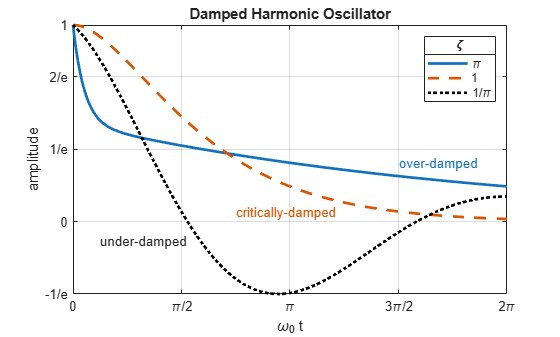# The Physics of the Damped Harmonic Oscillator

This example explores the physics of the damped harmonic oscillator by solving the equations of motion in the case of no driving forces. This example investigates the cases of under-, over-, and critical-damping.### Contents

1. Derive Equation of Motion

2. Solve the Equation of Motion (F = 0)

3. Underdamped Case ($\zeta <1$)

4. Overdamped Case ($\zeta >1$)

5. Critically Damped Case ($\zeta =1$)

6. Conclusion

### 1. Derive Equation of Motion

Consider a forced harmonic oscillator with damping shown below. Model the resistance force as proportional to the speed with which the oscillator moves.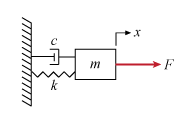Define the equation of motion where

• $m$ is the mass

• $c$ is the damping coefficient

• $k$ is the spring constant

• $F$ is a driving force

syms x(t) m c k F(t) eq = m*diff(x,t,t) + c*diff(x,t) + k*x == F
eq(t) =  

Rewrite the equation using $\mathit{c}=\mathit{m}\text{\hspace{0.17em}}\gamma$ and $\mathit{k}=\mathit{m}\text{\hspace{0.17em}}{\omega }_{0}^{2}$.

syms gamma omega_0 eq = subs(eq, [c k], [m*gamma, m*omega_0^2])
eq(t) =  

Divide out the mass $\mathit{m}$. Now we have the equation in a convenient form to analyze.

eq = collect(eq, m)/m
eq(t) =  

### 2. Solve the Equation of Motion where F = 0

Solve the equation of motion using dsolve in the case of no external forces where $F=0$. Use the initial conditions of unit displacement and zero velocity.

vel = diff(x,t); cond = [x(0) == 1, vel(0) == 0]; eq = subs(eq,F,0); sol = dsolve(eq, cond)
sol =  

Examine how to simplify the solution by expanding it.

sol = expand(sol)
sol =  

Notice that each term has a factor of ${\sigma }_{1}$, or ${e}^{-\gamma t/2}$, use collect to gather these terms

sol = collect(sol, exp(-gamma*t/2))
sol =  

The term $\sqrt{{\gamma }^{2}-4{\omega }_{0}^{2}}$ appears in various parts of the solution. Rewrite it in a simpler form by introducing the damping ratio $\zeta \equiv \frac{\gamma }{2{\omega }_{0}}$.

Substituting ζ into the term above gives:

$\sqrt{{\gamma }^{2}-4{\omega }_{0}^{2}}=2{\omega }_{0}\sqrt{{\left(\frac{\gamma }{2{\omega }_{0}}\right)}^{2}-1}=2{\omega }_{0}\sqrt{{\zeta }^{2}-1},$

syms zeta; sol = subs(sol, ... sqrt(gamma^2 - 4*omega_0^2), ... 2*omega_0*sqrt(zeta^2-1))
sol =  

Further simplify the solution by substituting $\gamma$ in terms of ${\omega }_{0}$ and $\zeta$,

sol = subs(sol, gamma, 2*zeta*omega_0)
sol =  

We have derived the general solution for the motion of the damped harmonic oscillator with no driving forces. Next, we'll explore three special cases of the damping ratio $\zeta$ where the motion takes on simpler forms. These cases are called

• underdamped $\left(\zeta <1\right)$,

• overdamped $\left(\zeta >1\right)$, and

• critically damped $\left(\zeta =1\right)$.

### 3. Underdamped Case ($\zeta <1$)

If $\zeta <1$, then $\sqrt{{\zeta }^{2}-1}=i\sqrt{1-{\zeta }^{2}}$ is purely imaginary

solUnder = subs(sol, sqrt(zeta^2-1), 1i*sqrt(1-zeta^2))
solUnder =  

Notice the terms ${\mathit{e}}^{\mathit{i}\text{\hspace{0.17em}}{\omega }_{0}\text{\hspace{0.17em}}\mathit{t}\sqrt{{\zeta }^{2}-1}}±\text{\hspace{0.17em}}{\mathit{e}}^{-{\mathit{i}\text{\hspace{0.17em}}\omega }_{0}\text{\hspace{0.17em}}\mathit{t}\sqrt{{\zeta }^{2}-1}}$ in the above equation and recall the identity ${\mathit{e}}^{\mathit{i}\text{\hspace{0.17em}}\mathit{x}}=\mathrm{cos}\left(\mathit{x}\right)+\mathit{i}\text{\hspace{0.17em}}\mathrm{sin}\left(\mathit{x}\right).$

Rewrite the solution in terms of $\mathrm{cos}$.

solUnder = coeffs(solUnder, zeta); solUnder = solUnder(1); c = exp(-omega_0 * zeta * t); solUnder = c * rewrite(solUnder / c, 'cos')
solUnder = ${\mathrm{e}}^{-{\omega }_{0} t \zeta } \mathrm{cos}\left({\omega }_{0} t \sqrt{1-{\zeta }^{2}}\right)$
solUnder(t, omega_0, zeta) = solUnder
solUnder(t, omega_0, zeta) = ${\mathrm{e}}^{-{\omega }_{0} t \zeta } \mathrm{cos}\left({\omega }_{0} t \sqrt{1-{\zeta }^{2}}\right)$

The system oscillates at a natural frequency of ${\omega }_{0}\sqrt{1-{\zeta }^{2}}$ and decays at an exponential rate of $1/{\omega }_{0}\phantom{\rule{0.16666666666666666em}{0ex}}\zeta$.

Plot the solution with fplot as a function of ${\omega }_{0}t$ and $\zeta$.

z = [0 1/4 1/2 3/4]; w = 1; T = 4*pi; lineStyle = {'-','--',':k','-.'}; fplot(@(t)solUnder(t, w, z(1)), [0 T], lineStyle{1}); hold on; for k = 2:numel(z) fplot(@(t)solUnder(t, w, z(k)), [0 T], lineStyle{k}); end hold off; grid on; xticks(T*linspace(0,1,5)); xticklabels({'0','\pi','2\pi','3\pi','4\pi'}); xlabel('t / \omega_0'); ylabel('amplitude'); lgd = legend('0','1/4','1/2','3/4'); title(lgd,'\zeta'); title('Underdamped');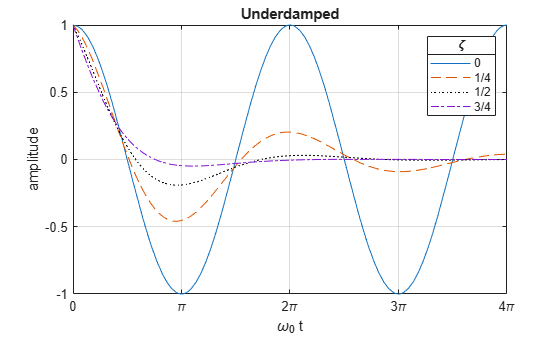### 4. Overdamped Case ($\zeta >1$)

If $\zeta >1$, then $\sqrt{{\zeta }^{2}-1}$ is purely real and the solution can be rewritten as

solOver = sol
solOver =  
solOver = coeffs(solOver, zeta); solOver = solOver(1)
solOver =  ${\mathrm{e}}^{-{\omega }_{0} t \zeta } \left(\frac{{\mathrm{e}}^{{\omega }_{0} t \sqrt{{\zeta }^{2}-1}}}{2}+\frac{{\mathrm{e}}^{-{\omega }_{0} t \sqrt{{\zeta }^{2}-1}}}{2}\right)$

Notice the terms $\frac{\left({\mathit{e}}^{{\omega }_{0}\mathit{t}\sqrt{{\zeta }^{2}-1}}+{\mathit{e}}^{-{\omega }_{0}\mathit{t}\sqrt{{\zeta }^{2}-1}}\right)}{2}$ and recall the identity $\mathrm{cosh}\left(\mathit{x}\right)=\frac{{\mathit{e}}^{\mathit{x}}+{\mathit{e}}^{-\mathit{x}}}{2}$.

Rewrite the expression in terms of $\mathrm{cosh}$.

c = exp(-omega_0*t*zeta); solOver = c*rewrite(solOver / c, 'cosh')
solOver = $\mathrm{cosh}\left({\omega }_{0} t \sqrt{{\zeta }^{2}-1}\right) {\mathrm{e}}^{-{\omega }_{0} t \zeta }$
solOver(t, omega_0, zeta) = solOver
solOver(t, omega_0, zeta) = $\mathrm{cosh}\left({\omega }_{0} t \sqrt{{\zeta }^{2}-1}\right) {\mathrm{e}}^{-{\omega }_{0} t \zeta }$

Plot the solution to see that it decays without oscillating.

z = 1 + [1/4 1/2 3/4 1]; w = 1; T = 4*pi; lineStyle = {'-','--',':k','-.'}; fplot(@(t)solOver(t, w, z(1)), [0 T], lineStyle{1}); hold on; for k = 2:numel(z) fplot(@(t)solOver(t, w, z(k)), [0 T], lineStyle{k}); end hold off; grid on; xticks(T*linspace(0,1,5)); xticklabels({'0','\pi','2\pi','3\pi','4\pi'}); xlabel('\omega_0 t'); ylabel('amplitude'); lgd = legend('1+1/4','1+1/2','1+3/4','2'); title(lgd,'\zeta'); title('Overdamped');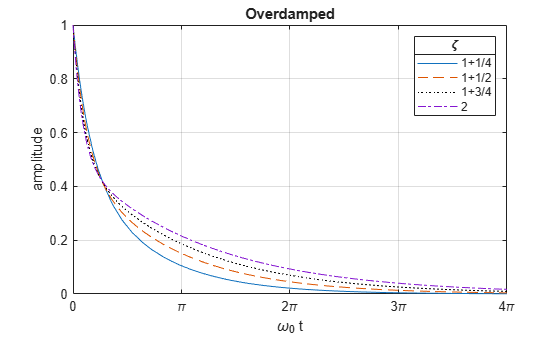### 5. Critically Damped Case ($\zeta =1$)

If $\zeta =1$, then the solution simplifies to

solCritical(t, omega_0) = limit(sol, zeta, 1)
solCritical(t, omega_0) = ${\mathrm{e}}^{-{\omega }_{0} t} \left({\omega }_{0} t+1\right)$

Plot the solution for the critically damped case.

w = 1; T = 4*pi; fplot(solCritical(t, w), [0 T]) xlabel('\omega_0 t'); ylabel('x'); title('Critically damped, \zeta = 1'); grid on; xticks(T*linspace(0,1,5)); xticklabels({'0','\pi','2\pi','3\pi','4\pi'});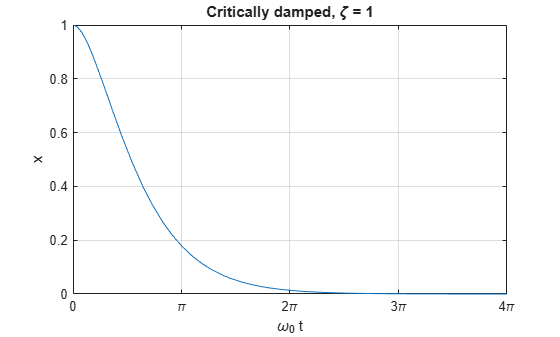### 6. Conclusion

We have examined the different damping states for the harmonic oscillator by solving the ODEs which represents its motion using the damping ratio $\zeta$. Plot all three cases together to compare and contrast them.

zOver = pi; zUnder = 1/zOver; w = 1; T = 2*pi; lineStyle = {'-','--',':k'}; fplot(@(t)solOver(t, w, zOver), [0 T], lineStyle{1},'LineWidth',2); hold on; fplot(solCritical(t, w), [0 T], lineStyle{2},'LineWidth',2) fplot(@(t)solUnder(t, w, zUnder), [0 T], lineStyle{3},'LineWidth',2); hold off; textColor = lines(3); text(3*pi/2, 0.3 , 'over-damped' ,'Color',textColor(1,:)); text(pi*3/4, 0.05, 'critically-damped','Color',textColor(2,:)); text(pi/8 , -0.1, 'under-damped'); grid on; xlabel('\omega_0 t'); ylabel('amplitude'); xticks(T*linspace(0,1,5)); xticklabels({'0','\pi/2','\pi','3\pi/2','2\pi'}); yticks((1/exp(1))*[-1 0 1 2 exp(1)]); yticklabels({'-1/e','0','1/e','2/e','1'}); lgd = legend('\pi','1','1/\pi'); title(lgd,'\zeta'); title('Damped Harmonic Oscillator');